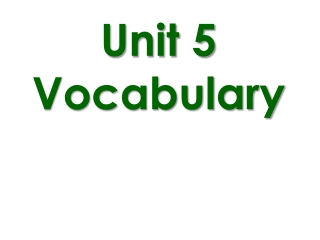DownloadDownload PresentationUnit 5 Vocabulary

# Unit 5 Vocabulary

Download Presentation## Unit 5 Vocabulary

- - - - - - - - - - - - - - - - - - - - - - - - - - - E N D - - - - - - - - - - - - - - - - - - - - - - - - - - -
##### Presentation Transcript

1. Unit 5 Vocabulary

2. Point An exact position or location in a given plane. A point has no dimension; it is represented by a dot. Point A or Point B

3. Line A line extends in one dimension. Represented by a straight line with two arrows to indicate that the line extends without end in two directions. There are an infinite number of points that continue beyond these points. Written as

4. Line Segment A line segment has two endpoints. Written as

5. Distance along a line The linear distance between two points on a given line.

6. Parallel Line Lines in a plane that either do not share any points and never intersect, or share all points. Written as

7. Perpendicular Line Two lines that intersect at a right angle (90°). Written as

8. Ray Part of a line that starts at a point and goes off into one direction infinitely.

9. Angle A shape, formed by two lines or rays diverging from a common point (the vertex). The angle is

10. Right Angle An angle that measures 90°.

11. Acute Angle An angle measuring less than 90° but greater than 0°.

12. Obtuse Angle An angle measuring greater than 90° but less than 180°.

13. Circle • The set of all points in a plane equidistant from a certain point called the center.

14. One-to-One A relationship wherein each point in a set of points is mapped to exactly one other point.

15. Pre-image The original figure before undergoing a transformation.

16. Image The new, resulting figure after a transformation

17. Isometry A transformation in which the preimage and image are congruent.

18. Transformations are called RIGID if every image is congruent to its preimage. Rigid transformations can also be referred to as an ISOMETRY. Every segment is congruent to its image.

19. Which of the following are rigid transformations? (Isometry)

20. Isometries not onlypreserve lengths, butthey preserve angle measuresparallel lines, andbetweenness of points

21. Find the value of each variable, given that the transformation is an isometry.

22. Congruent Figures are congruent if they have the same shape, size, lines, and angles.

23. Similar Triangles Triangles are similar if they have the same shape but have different sizes.

24. Homework AssignmentPractice WS